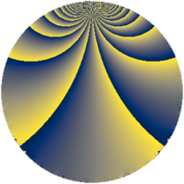# Properties

 Label 230.3.iLevel $230$ Weight $3$ Character orbit 230.i Rep. character $\chi_{230}(19,\cdot)$ Character field $\Q(\zeta_{22})$ Dimension $240$ Newform subspaces $1$ Sturm bound $108$ Trace bound $0$

# Related objects

## Defining parameters

 Level: $$N$$ $$=$$ $$230 = 2 \cdot 5 \cdot 23$$ Weight: $$k$$ $$=$$ $$3$$ Character orbit: $$[\chi]$$ $$=$$ 230.i (of order $$22$$ and degree $$10$$) Character conductor: $$\operatorname{cond}(\chi)$$ $$=$$ $$115$$ Character field: $$\Q(\zeta_{22})$$ Newform subspaces: $$1$$ Sturm bound: $$108$$ Trace bound: $$0$$

## Dimensions

The following table gives the dimensions of various subspaces of $$M_{3}(230, [\chi])$$.

Total New Old
Modular forms 760 240 520
Cusp forms 680 240 440
Eisenstein series 80 0 80

## Trace form

 $$240q + 48q^{4} - 8q^{6} + 96q^{9} + O(q^{10})$$ $$240q + 48q^{4} - 8q^{6} + 96q^{9} + 154q^{15} - 96q^{16} + 44q^{20} + 16q^{24} - 84q^{25} + 32q^{26} - 100q^{29} - 352q^{30} + 124q^{31} + 28q^{35} - 192q^{36} + 72q^{39} + 116q^{41} - 148q^{46} - 188q^{49} + 144q^{50} + 324q^{54} + 796q^{55} - 264q^{56} + 400q^{59} + 176q^{60} - 616q^{61} + 192q^{64} + 462q^{65} - 176q^{66} + 120q^{69} - 504q^{70} + 464q^{71} - 528q^{74} - 934q^{75} - 968q^{79} - 264q^{80} + 664q^{81} - 352q^{84} - 1196q^{85} + 396q^{86} + 376q^{94} + 126q^{95} - 32q^{96} - 3300q^{99} + O(q^{100})$$

## Decomposition of $$S_{3}^{\mathrm{new}}(230, [\chi])$$ into newform subspaces

Label Dim. $$A$$ Field CM Traces $q$-expansion
$$a_2$$ $$a_3$$ $$a_5$$ $$a_7$$
230.3.i.a $$240$$ $$6.267$$ None $$0$$ $$0$$ $$0$$ $$0$$

## Decomposition of $$S_{3}^{\mathrm{old}}(230, [\chi])$$ into lower level spaces

$$S_{3}^{\mathrm{old}}(230, [\chi]) \cong$$ $$S_{3}^{\mathrm{new}}(115, [\chi])$$$$^{\oplus 2}$$